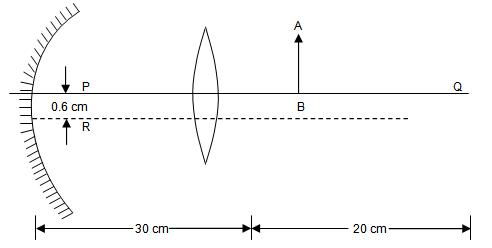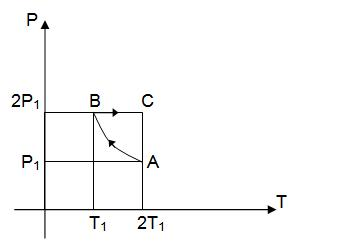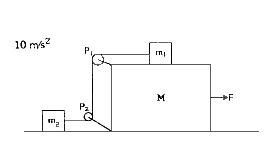Click to Chat

1800-1023-196

+91-120-4616500

CART 0

• 0

MY CART (5)

Use Coupon: CART20 and get 20% off on all online Study Material

ITEM
DETAILS
MRP
DISCOUNT
FINAL PRICE
Total Price: Rs.

There are no items in this cart.
Continue Shopping• Complete JEE Main/Advanced Course and Test Series
• OFFERED PRICE: Rs. 15,900
• View Details

```IIT-JEE-Physics-Mains–2000

MAINS

Time : two hours                                                                 Max. Marks : 100
______________________________________________________________________

General Instructions :
1. There are ten questions in this paper. Attempt all Questions.
2. Answer each question starting on a new page. The corresponding question number must be written in the left margin. Answer all the parts of a question at one place only.
3. Use only Arabic numerals (0, 1, 2 ………….9) in answering the questions irrespective of the language in which your answer.
4. Use of logarithmic tables is not permitted.
5. Use of calculator is not permitted.
______________________________________________________________________

PART B
1. (a) A hydrogen like atom of atomic number Z is in an excited state of quantum number 2n. It can emit a maximum energy photon of 204 eV. If it makes a transition to quantum state n, a photon of energy 40.8 eV is emitted. Find n, Z and the ground state energy (in eV) of this atom. Also calculate the minimum energy (in eV) that can be emitted by this atom during de-excitation. Ground state energy of hydrogen atom is –13.6 eV.
(b) When a beam of 10.6 eV photons of intensity 2.0 W/m2 falls on a platinum surface of area 1.0 × 10-4 m2 and work function 5.6 eV, 0.53% of the incident photons eject photoelectrons. Find the number of photoelectrons emitted per second and their minimum and maximum energies (in eV). Take 1eV = 1.6 × 10-19 J.

2. (a) A convex lens of focal length 15 cm and a concave mirror of focal length 30 cm are kept with their optic axis PQ and RS parallel but separated in vertical direction by 0.6 cm as shown. The distance between the lens and mirror is 30 cm. An upright object AB of height 1.2 cm is placed on the optic axis PQ of the lens at a distance of 20 cm from the lens. If A’ B’ is the image after refraction from the lens and thereflection from the mirror, find the distance of A’ B’ from the pole of the mirror and obtain its magnification. Also locate positions of A’ and B’ with respect to the optic axis RS.
(b) A glass plate of refractive index 1.5 is coated with a thin layer of thickness t and refractive index 1.8. Light of wavelength l travelling in air is incident normally on the layer. It is partly reflected at the upper and the lower surfaces of the layer and the two reflected rays interfere. Write the condition for their constructive interference constructively.

3. A 3.6 m long pipe resonates with a source of frequency 212.5 Hz when water level is at certain heights in the pipe. Find the heights of water level (from the bottom of the pipe) at which resonances occur. Neglect end correction. Now the pipe is filled to a height H (»3.6 m). A small hole is drilled very close to its bottom and water is allowed to leak. Obtain an expression for the rate of fall of water level in the pipe as a function of H. If the radii of the pipe and the hole are 2 × 10–2 m and 1 × 10–3 m respectively, calculate the time interval between the occurrence of first two resonances. Speed of sound in air is 340 m/s and g = 10 m/s2.

4. Two moles of an ideal monoatomic gas is taken through a cycle ABCA as shown in the P–T diagram. During the process AB, pressure and temperature of the gas vary such that PT = constant. If T1 = 300 K, calculate(A) the work done on the gas in the process AB and

(B) the heat absorbed or released by the gas in each of the processes
Give answers in terms of the gas constant R.

5. A thermocole vessel contains 0.5 Kg of distilled water at 30oC. A metal coil of are 5 × 10–3 m2, number of turns 100, mass 0.06 Kg and resistance 1.6 W is lying horizontally at the bottom of the vessel. A uniform time varying magnetic field is set up to pass vertically through the coil at time t = 0. The field is first increased from zero to 0.8 T at a constant rate between 0 and 0.2s and then decreased to zero at the same rate between 0.2 and 0.4s. The cycle is repeated 12000 times. Make sketches of the current through the coil and the power dissipated in the coil as a function of time for the first two cycles. Clearly indicate the magnitudes of the quantities on the axes. Assume that no heat is lost to the vessel or the surroundings. Determine the final temperature of the water under thermal equilibrium. Specific heat of metal = 500 J/Kg–K and the specific heat of water = 4200 J/Kg–K. Neglect the inductance of coil.

6. Four point charges + 8 mC, – 1 mC and + 8 mC are fixed at the points -√(27/2) m,-√(3/2) m,+√(3/2) m and+√(27/2) m respectively on the y-axis. A particle of mass 6 × 10-4 Kg and charge + 0.1mC moves along the –x direction. Its speed at x = + ¥ is V0. Find the least values of V0 for which the particle will cross the origin. Find also the kinetic energy of the particle at the origin. Assume that space is gravity free. 1/4p Î0 = 9 × 109 Nm2/C2.

7. A circular loop of radius R is bent along aType equation here. diameter and given a shape as shown in figure. One of the semicircles (KNM) lies in the x-z plane and the other one (KLM) in the y-z plane with their centres at origin. Current I is flowing through each of the semicircles as shown in figure.(a) A particle of charge q is released at the origin with a velocity vector V=-V0 î.
Find the instantaneous force vector F on the particle. Assume that space is gravity free.

(b) If an external uniform magnetic field B0 j is applied, determine the force vectors F1 &  F2 on the semicircles KLM and KNM due to the field and the net force vector F on the loop.

8. An object A is kept fixed at the point x = 3 m and y = 1.25 m on a plank P raised above the ground. At time t = 0 the plank starts moving along the + xdirection with an acceleration 1.5 m/s2. At the same instant a stone is projected from the origin with a velocity vector u as shown. A stationary person on the ground observes the stone hitting the object during its downward motion at an angle of 45o to the horizontal. All the motions are in X-Y plane. Find vector u and the time after which the stone hits the object. Take g = 10 m/s2.

9. A rod AB of mass M and length L is lying on a horizontal frictionless surface. A particle of mass m travelling along the surface hits the end ‘A’ of the rod with a velocity V0 in a direction perpendicular to AB. The collision is elastic. After the collision the particle comes to rest.
(a) Find the ratio m/M.
(b) A point P on the rod is at rest immediately after collision. Find the distance AP.
(c) Find the linear speed of the point P after a time pL/3V0 after the collision.

10. In the figure masses m1, m2 and M are 20 Kg, 5 Kg and 50 Kg respectively. The coefficient of friction between M and ground is zero. The coefficient of friction between m1 and M and that between m2 and ground is 0.3. The pulleys and the strings are massless. The string is perfectly horizontal between P1 and m1 and also between P2 and m2. The string is perfectly vertical between P1 and P2. An external horizontal force F is applied to the mass M. Take g = 10 m/s2.(a) Draw a free body diagram of mass M, clearly showing all the forces.
(b) Let the magnitude of the force of friction between m1 and M be f1 and 2f2. Find f1 and f2. Write equations of motion of all the masses. Find F, tension in the string and accelerations of the masses.
```### Course Features

• 731 Video Lectures
• Revision Notes
• Previous Year Papers
• Mind Map
• Study Planner
• NCERT Solutions
• Discussion Forum
• Test paper with Video Solution